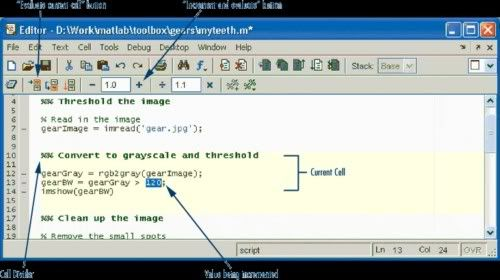May 24

## How to Generate Report Automatically From Matlab

Posted by abiao at 15:39 | Code » Matlab | Comments(8) | Reads(22675)
Often we have to generate reports to our boss, colleagues, and clients, etc. Generally those reports include Matlab source codes, maths equations, and possibly graphs. How do you generate your reports? writing down your equations in Word, running your matlab codes, copying and pasting the results?It is all right to do like that, but the whole process becomes extremely simple with the cell mode in Matlab, it generates report automatically for us, any change you make regarding description, codes and results will be updated by clicking a simple button: Publish to HTML.

The following steps outline the procedure,
First, opening an editor in Matlab by choosing File -> New -> M-file;
Second, selecting cell and enable cell mode in the editor;
Third, inserting cell divider under cell window, it allows you to type a name for each section, similar with the title for chapters in Word;
Fourth, depending on your reports, inserting Text Markup, where you are able to insert description text, bold text, etc. most importantly, it supports TeX equations;
Finally, clicking "Publish to HTML", a nice-looking report is generated, and if necessary, changing the codes and re-running to update the report.

For a simple demonstration, I write the files as follows.
%% Cell mode publish demonstration
% abiao @ mathfinance.cn

%% Using Black Scholes formula as an example
% The value of a call and put options in terms of the Black–Scholes parameters
% is:
%
% $$C(S,t) = SN(d_1) - Ke^{-r(T - t)}N(d_2) \,$$
%
% $$P(S,t) = Ke^{-r(T-t)} - S + (SN(d_1) - Ke^{-r(T - t)}N(d_2)) = Ke^{-r(T-t)} - S + C(S,t)$$
%
% $$d_1 = \frac{\ln(\frac{S}{K}) + (r + \frac{\sigma^2}{2})(T - t)}{\sigma\sqrt{T - t}}$$
%
% $$d_2 = d_1 - \sigma\sqrt{T - t}$$

%% Sample code, for simplicity, I use the embedded command
[call, put] = blsprice(10,9,0.02,2,0.3);
fprintf('Call option value is %g. \n',call)
fprintf('Put option value is %g. \n',put)
call1 = [];
put1 = [];
for i = 1:10
call1(i) = blsprice(5+i,9,0.02,2,0.3);
end

%% Sample plot for demonstration only
plot(5+(1:10), call1)

The final report is shown at http://www.mathfinance.cn/attachment/CellPublishDemo.html, looks fantastic.

Tags:
Great functions!!

btw, the new theme is simple and nice, except for the huge Citi banner on the toplittle bit wierd...
Thanks for your compliment and support, nychole. Regarding the banner, I will think about re-position it.How many reports are there? I need some of them so that I can learn something about assignment service and I need to do it asap. It will help me to know the things that we are going to work on.
[b].  Thanks for sharing amazing information!!!!!!
 Add a comment Emots Enable HTML Enable UBB Enable Emots Hidden Remember Nickname   Password   Optional Site URI   Email   [Register]